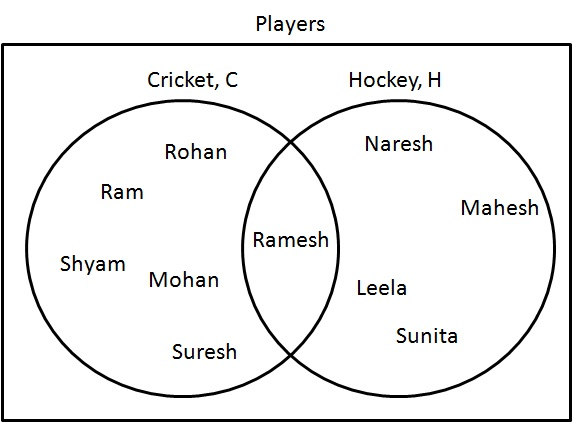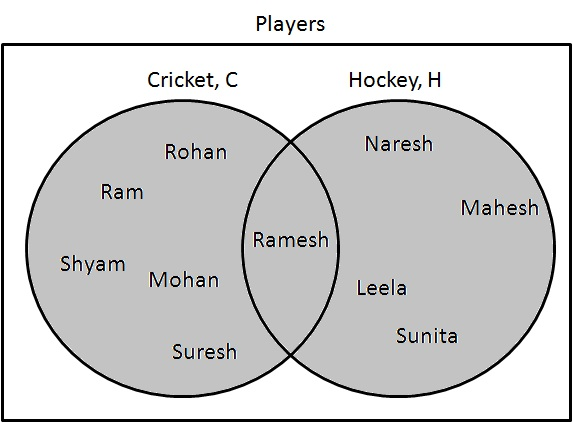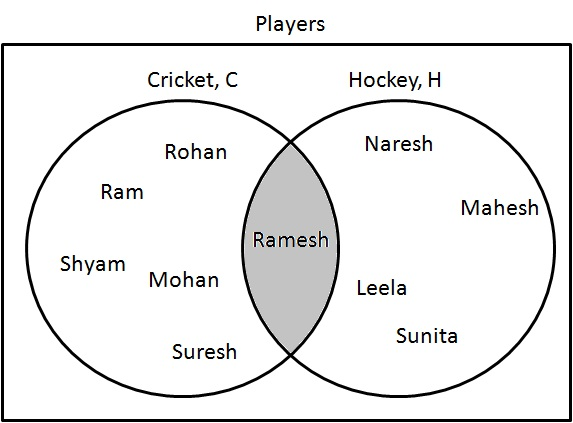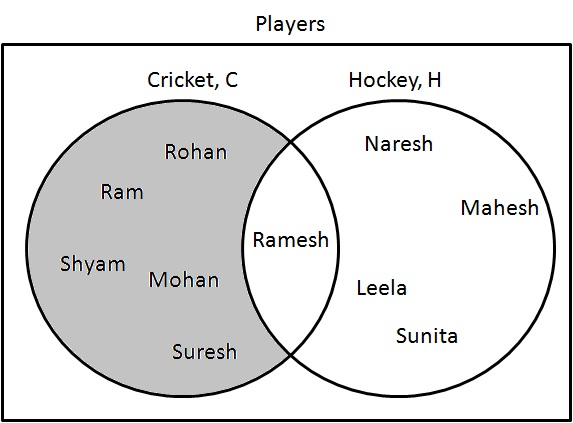# Statistics - Venn Diagram

Venn diagram is a way to visually represents relationship between groups of entities or objects. Venn diagrams are comprised of circles where each circle represents a whole set. Venn diagram can have unlimited circles but generally two or three circles are preferred otherwise the diagram becomes too complex.

## Steps to draw a Venn Diagram

Consider the following sets of people:

1. Cricket Players - $C = \{ Ram, Shyam, Mohan, Rohan, Ramesh, Suresh \}$

2. Hockey Players - $H = \{ Ramesh, Naresh, Mahesh, Leela, Sunita \}$

Step 1: Draw a rectangle and label it as players.

Step 2: Draw two circles and label them as Cricket and Hockey. Make sure that circles are overlapping each other.

Step 3: Write Names inside the circle as relevant. Common name(s) should fall within common region.## Union

Union ($\cup$) represents a set where items are present in all categories but are not repeated.

### Example

Problem Statement:

Draw a Venn diagram of $C \cup H$.

Solution:

Step 1: Determine players who are either playing cricket or hockey. Draw them as following:

$C \cup H = \{ Ram, Shyam, Mohan, Rohan, Ramesh, Suresh, Naresh, Mahesh, Leela, Sunita \}$.## Intersection

Intersection ($\cap$) represents a set where items are present in both categories.

### Example

Problem Statement:

Draw a Venn diagram of $C \cap H$.

Solution:

Step 1: Determine players who are playing cricket and hockey both. Draw them as following:

$C \cap H = \{ Ramesh \}$.## Difference

Difference ($-$) represents a set where items are present only in one category and not in other one.

### Example

Problem Statement:

Draw a Venn diagram of $C - H$.

Solution:

Step 1: Determine players who are playing cricket only. Draw them as following:

$C - H = \{ Ram, Shyam, Mohan, Rohan, Suresh \}$.